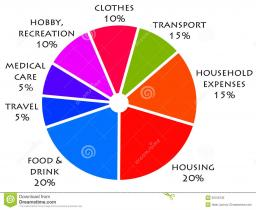# Expenses 2582

The family pays 250 euros a month for food, which is 33% of the total budget. How much money do they have left for other expenses?

x =  507.58 eur

### Step-by-step explanation:

0.33 y = 250
x = y - 250

0.33·y = 250
x = y - 250

0.33y = 250
x-y = -250

Pivot: Row 1 ↔ Row 2
x-y = -250
0.33y = 250

y = 250/0.33 = 757.57575758
x = -250+y = -250+757.57575758 = 507.57575758

x = 16750/33 ≈ 507.575758
y = 25000/33 ≈ 757.575758

Our linear equations calculator calculates it.Did you find an error or inaccuracy? Feel free to write us. Thank you!

Tips for related online calculators